Home

• Access
• Open access
• Cited by 2

### Actions:

MathJax
MathJax is a JavaScript display engine for mathematics. For more information see http://www.mathjax.org.
• # Send article to Kindle

Note you can select to send to either the @free.kindle.com or @kindle.com variations. ‘@free.kindle.com’ emails are free but can only be sent to your device when it is connected to wi-fi. ‘@kindle.com’ emails can be delivered even when you are not connected to wi-fi, but note that service fees apply.

Find out more about the Kindle Personal Document Service.

Long-pulse, single-frequency 1064 nm laser and frequency doubling
Available formats
×

# Send article to Dropbox

To send this article to your Dropbox account, please select one or more formats and confirm that you agree to abide by our usage policies. If this is the first time you use this feature, you will be asked to authorise Cambridge Core to connect with your <service> account. Find out more about sending content to Dropbox.

Long-pulse, single-frequency 1064 nm laser and frequency doubling
Available formats
×

# Send article to Google Drive

To send this article to your Google Drive account, please select one or more formats and confirm that you agree to abide by our usage policies. If this is the first time you use this feature, you will be asked to authorise Cambridge Core to connect with your <service> account. Find out more about sending content to Google Drive.

Long-pulse, single-frequency 1064 nm laser and frequency doubling
Available formats
×

## Abstract

An all-solid-state single-frequency 1064 nm laser with a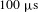$100~{\rm\mu}\text{s}$ pulse width, 500 Hz repetition rate and 700 mJ single pulse energy is designed using seed injection and a three-stage master oscillator power amplifier (MOPA) construction. Using this as a basis, research on long-pulse laser frequency doubling is carried out. By designing and optimizing the lithium triborate (LBO) crystal, the theoretically calculated maximum conversion efficiency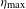${\it\eta}_{\max }$ reaches 68% at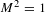$M^{2}=1$ , while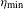${\it\eta}_{\min }$ is 33% at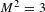$M^{2}=3$ . Generation of 212 mJ pulses of green light with a repetition rate as high as 500 Hz is obtained from a fundamental energy of 700 mJ. The experimental conversion efficiency reaches 31% and the power stability is better than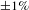$\pm 1\%$ .

## 1. Introduction

In recent years, with the development of adaptive optics, quantum optics and optical communications, high-power all-solid-state lasers are required[1, 2]. Single-frequency lasers are among the most important and active research fields owing to their good beam quality, long coherence length and narrow spectral linewidth[3, 4]. In particular, as a high-repetition-frequency, long-pulse single-frequency green light laser with an ultra-narrow linewidth can measure distance by time gating, it has special applications in the field of astronomical observations; moreover, it has significant advantages in the field of laser processing compared with short-pulse lasers.Figure 1. Schematic diagram of the high-repetition-rate, long-pulse, single-frequency seed laser.Figure 2. Schematic diagram of the MOPA construction.

At present, twisted-mode cavities, short cavities and ring cavities are the proven methods to obtain single-frequency lasers. The first two methods are mainly applied in low-power all-solid-state single-frequency lasers. The ring cavity can achieve higher power operation, but the oscillation of multiple longitudinal modes and mode skipping are liable to occur, and astigmatism is difficult to avoid. Thanks to the mature technology of single-frequency CW fibre lasers, it is a perfect way to achieve high-repetition-frequency, high-energy single-frequency laser pulses by injecting an externally modulated single-frequency CW seed laser into the following mixed fibre and solid-state laser amplifier. The mixed amplifier structure has some unique advantages: (i) the temporal profile is smooth, the pulse width and frequency repetition rate are easy to control and the central wavelength is tunable. (ii) Both the single-frequency features of the highly elliptical core fibre laser amplifier and the high extraction efficiency and good beam quality of the solid-state laser amplifier are maintained. (iii) Stimulated scattering and amplified spontaneous emission (ASE), which are easily caused in short-pulse and high-repetition-rate fibre laser amplifiers, are avoided.

An efficient way to obtain a green laser is by nonlinear frequency conversion[11, 12]. Intra-cavity frequency doubling can take advantage of the higher power density of the fundamental light in the cavity to achieve a high conversion efficiency and low pump threshold, but the beam quality is difficult to control. In comparison, extra-cavity frequency doubling is a better choice for the mentioned seed light injection amplification structure since the optical–optical efficiency is high and the beam quality is easily controlled.

In this paper, a single-frequency long-pulse seed laser (with a pulse length of a hundred microseconds) is obtained by externally modulating a super-narrow spectrum linewidth, single-frequency CW fibre laser. After injecting the seed laser into a series of solid-state amplifiers, a 1064 nm long-pulse single-frequency laser is achieved with a high repetition frequency, high power and high beam quality. In addition, based on frequency doubling this fundamental 1064 nm laser, a green laser with an pulse output of 212 mJ is achieved. There have been no similar green lasers reported so far, but in 2006 Yarrow reported on a 1064 nm single-frequency laser with a long pulse duration and an average power of 11.3 W. In 2012, Wang demonstrated a high-energy, single-frequency long-pulse laser with a hundred microsecond pulse length, a pulse energy of 180 mJ, a repetition rate of 50 Hz and a pulse width of$150~{\rm\mu}\text{s}$ in 2012. Lu reported a high-energy all-solid-state sodium beacon laser with a pulse energy of 380 mJ at a repetition rate of 50 Hz with a pulse width of$150~{\rm\mu}\text{s}$[9, 14, 15]. To the best of our knowledge, this is the highest power yet achieved for a long-pulse, single-frequency green laser with a repetition rate of 500 Hz.Figure 3. SHG efficiency of (a) an ideal Gaussian laser beam ($M^{2}=1$) and (b) a non-perfect Gaussian laser beam ($M^{2}=3$).

## 2. High-repetition-rate 1064 nm single-frequency laser

The primary problem in developing an all-solid-state single-frequency laser with a high repetition rate, high energy and high beam quality is to obtain a long-pulse single-frequency seed laser with an easily controlled wavelength. The scheme to obtain the seed laser is shown in Figure 1. The single-frequency CW seed source is a high-quality distributed feedback laser, with an output power of 10 mW and a linewidth of less than 5 kHz. Combined with high-precision wavelength control technology, precise stability control can be implemented, giving a tuning range for the central wavelength from 1064.3 to 1065.0 nm. With the help of the fibre amplifier, the output power of the single-frequency CW seed source can reach 7 W. After the collimator, isolation and electro optic modulator (EOM), a 500 Hz, 0.7 mJ single-frequency pulse laser with a standard rectangular waveform is obtained. On reflection at the Brewster angle on the polarizer and injection into the subsequent amplifier, the seed source can reach a very high degree of polarization.

As the energy of pulse seed laser is only 0.7 mJ, multi-stage amplification is necessary to obtain a hundreds of millijoule laser. Due to the severe thermal effects of the high-repetition-rate amplification system, the operating current should not be too high. Considering all the requirements – laser output energy, beam quality, system complexity and other factors – a three-stage master oscillator power amplifier (MOPA) system is proposed.

As shown in Figure 2, the first stage is a double-pass amplifier, including two 25-bar LD-pumped Nd:YAG modules. The pumping structure of the modules is cyclic, consisting of five tori with five LD arrays around the circumference, and the diameter of the Nd:YAG rod is 4 mm. The peak pump power of the module is 3800 W, with a pump pulse width of$250~{\rm\mu}\text{s}$, which is$160~{\rm\mu}\text{s}$ ahead of the seed pulse in the time domain. Considering the requirements for the output energy and control of the beam quality, the second-stage amplifier also uses two 25-bar LD-pumped Nd:YAG modules, while the amplifier is a single-pass design. The pump pulse width is again$250~{\rm\mu}\text{s}$, which is$170~{\rm\mu}\text{s}$ ahead of the seed pulse. The third-stage amplifier is the main amplifier, where, to control the beam quality, four 54-bar LD-pumped single-pass Nd:YAG modules are proposed to reduce thermal effects, and$90^{\circ }$ quartz rotators and 4f imaging systems are used to compensate the depolarization loss caused by the birefringence. The module is cyclic with a 9-6 annular pumping structure surrounding a Nd:YAG rod of diameter 10 mm. The peak input pump power of the module is 5300 W with a pump pulse width of$250~{\rm\mu}\text{s}$, which is$180~{\rm\mu}\text{s}$ ahead of the seed pulse in the time domain. The LDs of the different stages are triggered at different times in order to achieve better coincidence of the incident laser pulse and the drive power pulse in the time domain – in such a case, the maximum output laser power is obtained and the relaxation oscillation peak is weakened.

## 3. Long-pulse single-frequency green laser

It is feasible to obtain a conversion efficiency of more than 50% in frequency doubling with a short-pulse fundamental laser. But the conversion efficiency decreases rapidly, as the peak power is much lower in the long-pulse fundamental laser condition. The situation can be improved by increasing the length of the frequency-doubling crystal, but in turn the angle mismatch factor and temperature mismatch factor are also increased, which will limit any further improvement of efficiency and destroy the system stability. It will be advantageous for obtaining a high conversion efficiency to focus the incident laser, because the power density is enhanced as the beam spot size gets smaller. However, the Rayleigh length will also get shorter, which will decrease the conversion efficiency. Therefore, to obtain a high conversion efficiency, we should take into account the combined effects of the crystal length, the phase mismatch, the incident laser aperture and the focusing properties.

Furthermore, the nonlinear crystal should have low absorption for both the fundamental laser and the frequency-doubled laser, because absorption of the fundamental laser in the crystal may produce large thermal gradients, which will result in a temperature mismatch, or even damage to the nonlinear crystal. Taking all these factors into consideration, a lithium triborate (LBO) crystal working in type I noncritical phase matching at a temperature of$149\,^{\circ }\text{C}$ is an ideal choice. At this temperature, the absorption coefficients of both the fundamental and the harmonic laser are less than 50 ppm, and the reception parameters (angle, linewidth, temperature, etc.) are large.

In addition, beam quality is an important factor affecting the frequency-doubling efficiency of the long-pulse laser – we can obtain a smaller focused laser spot size and a higher intensity with better beam quality from the same incident conditions and focusing system. Following the model in Ref. , the conversion efficiency with different beam quality factors ($M^{2}=1\text{ and }3$) is calculated, using the same incident energy (700 mJ), pulse width ($100~{\rm\mu}\text{s}$), crystal length (50 mm) and phase matching type (type I). The results are shown in Figures 3 and 4, respectively. This model takes full consideration of the influence of the crystal length and the coupling incidence conditions.

As shown in Figure 3(a), we get the highest conversion efficiency (68%) in the case of beam quality$M^{2}=1$. However, this falls to 33% with beam quality$M^{2}=3$, as shown in Figure 3(b). Accordingly, the beam quality of the fundamental laser has a significant impact on the frequency-doubling efficiency, so we should try to ensure good beam quality in the fundamental laser as well as a low level of aberrations induced by the coupling focusing system.

## 4. Results and analysis

Figure 4 shows the laser power and beam quality ($M^{2}$) of each amplifier on injecting the 500 Hz, 0.7 mJ seed laser. The first stage amplifies the 0.7 mJ seed laser to 45 mJ (a amplification factor of 65) – which can be considered as small-signal amplification – and the beam quality$M^{2}$ is 1.2. The output of second stage is 140 mJ (an amplification factor of 3.1) and$M^{2}$ is 1.4.Figure 4. Output laser power and beam quality ($M^{2}$) of each amplifier.

The third amplifier is the main amplifier, giving an output energy of 700 mJ (an amplification factor of 5). The temporal profiles of the pump and final output laser pulse are shown in Figure 5. The laser pulse is smooth, with a full-width at half-maximum (FWHM) of$100~{\rm\mu}\text{s}$. The large diameter of the laser rod (${\it\Phi}=10~\text{mm}$) leads to serious spherical aberration and poor beam quality as a result. A 4f system comprising a pair of positive lens is applied to compensate the spherical aberration, the focal length of the lenses used is 200 mm and the image plane is the principal plane of the thermal lens. This 4f system improves the beam quality$M^{2}$ from 4.8 to 2.8.Figure 5. Waveform of the output pulse from the main amplifier.

The short fibre delayed self-heterodyne method is used to measure the linewidth of the single-frequency laser. The results are shown in Figure 6. The measured linewidth is 37.4 kHz, i.e. the actual value is 18.7 kHz according to the measuring principle, which is half of the measured value.Figure 6. Linewidth of main amplifier output laser measurement.

The experiment on the long-pulse single-frequency green laser is carried out using the injected fundamental laser of pulse power 700 mJ – the beam quality$M^{2}=2.8$ and the beam spot size is 5 mm before the focusing lens ($f=250~\text{mm}$). The temperature of the nonlinear crystal LBO is precisely controlled in an oven with a control accuracy of$\pm 0.05\,^{\circ }\text{C}$ and the damage threshold of the crystal is higher than$18~\text{GW}/\text{cm}^{2}$ in order to meet the high peak power requirement of the fundamental laser. The power obtained for the green laser is 212 mJ, with a conversion efficiency reaching 31%, which agrees well with the results of theoretical calculations. The measured beam quality of the green laser is shown in Figure 7(a), the results being$M_{x}^{2}=3.41$,$M_{y}^{2}=3.62$.Figure 7. (a) Beam profile and$M^{2}$ of the single-frequency green laser, and (b) far-field and (c) near-field beam profiles of the fundamental laser.

The stability of the output power and the green laser wavelength are measured over a period of 15 min. Thanks to the excellent stability of the fundamental laser and the nonlinear process, the root mean square (RMS) power stability is better than$\pm 0.5\%$, with a peak-to-valley (PV) value of better than$\pm 1\%$. Because of the superior features of the fundamental seed laser, the PV value of wavelength is better than$\pm 0.04~\text{pm}$ and the linewidth of the green laser is 37 kHz. Furthermore, the wavelength is tunable from 532.15 to 532.50 nm.

## 5. Conclusions

By injecting the long laser pulse (with a duration of hundreds of microseconds) obtained by externally modulating a single-frequency CW fibre laser into multi-stage amplifiers, a 1064 nm single-frequency laser with a linewidth of 18.7 kHz is achieved. The single-shot energy is 700 mJ, with a pulse width of$100~{\rm\mu}\text{s}$, a repetition rate of 500 Hz, and a beam quality$M^{2}=2.8$. In the next stage, we carry out an investigation of a green laser based on frequency doubling that 1064 nm single-frequency laser. The output of the single-frequency green laser is 212 mJ, with a beam quality of$M^{2}=3.5$, a linewidth of 37 kHz, and a conversion efficiency higher than 30%.

## Acknowledgement

This research was supported by the Technology Development Foundation of China Academy of Engineering Physics (2012A0401020).

## References

1.Zhou, S. Zhao, H. and Tang, X. Chin. J. Lasers 36, 1605 (2009).
2.Azcárate, M. L. Codnia, J. Diodati, F. P. Hnilo, A. A. and Larotonda, M. A. Proc. SPIE 3572, 370 (1999).
3.Hovis, F. E. Edelman, J. Schum, T. Rudd, J. and Andes, K. Proc. SPIE 6871, 68710E (2008).
4.Fan, T. Y. IEEE J. Quantum Electron. 3, 11 (2005).
5.Evtuhov, V. and Siegman, A. E. Appl. Opt. 4, 1 (1965).
6.Zayhowski, J. J. and Mooradian, A. Opt. Lett. 14, 1 (1989).
7.Martin, K. I. Clarkson, W. A. and Hanna, D. C. Opt. Lett. 21, 12 (1996).
8.Ostermeyer, M. Kappe, P. Menzel, R. and Wulfmeyer, V. Appl. Opt. 4, 44 (2005).
9.Yarrow, M. J. Kim, J. W. and Clarkson, W. A. Opt. Commun. 270, 361 (2007).
10.Dong, X. Xiao, H. Xu, S. Pan, Z. Ma, Y. Wang, X. Zhou, P. and Yang, Z. Chin. Opt. Lett. 9, 111404 (2011).
11.Li, P. Li, G. Chen, M. Zhang, D. and Huang, D. Proc. SPIE 7382, 738252 (2009).
12.Zhang, H. Zhang, Y. Tian, X. Geng, Y. Zhong, K. Li, X. Wang, P. and Yao, J. Chin. Opt. Lett. 7, 802 (2009).
13.Boyd, R. W. Nonlinear Optics (Elsevier, 2008).
14.Wang, C. Zhang, X. Ye, Z. Liu, C. and Chen, J. Appl. Opt. 52, 19 (2013).
15.Lu, Y.-H. Xie, G. Zhang, L. and Fan, G.-B. Appl. Phys. B 112, 2 (2015).